# AP Chemistry Chapter 7 Atomic Structure and Periodicity

• Slides: 41AP Chemistry Chapter 7 Atomic Structure and Periodicity Expected Test Date Thursday Feb 5 th-6 thWhat is Atomic Structure and Periodicity? �Evidence of the atomic structure �How groups and periods show similar properties �Most of this has to do with the arrangement of atoms in an element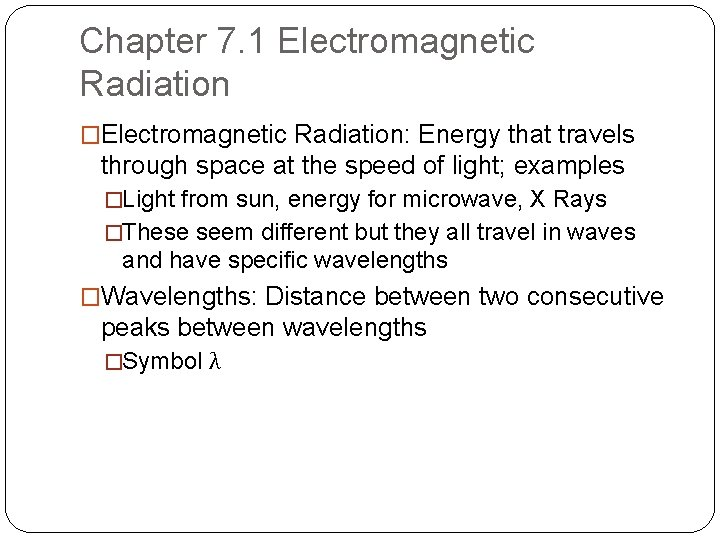Chapter 7. 1 Electromagnetic Radiation �Electromagnetic Radiation: Energy that travels through space at the speed of light; examples �Light from sun, energy for microwave, X Rays �These seem different but they all travel in waves and have specific wavelengths �Wavelengths: Distance between two consecutive peaks between wavelengths �Symbol λChapter 7. 1 Electromagnetic Radiation �Frequency: Number of wavelengths per second �Symbol v �EQUATION TO REMEMBER c = λv �Given to you on ap exam formula sheet �c = speed of light which is 2. 998 X 10 ms-1 �Speed of light also given to you on AP Chemistry formula sheetChapter 7. 1 Electromagnetic Radiation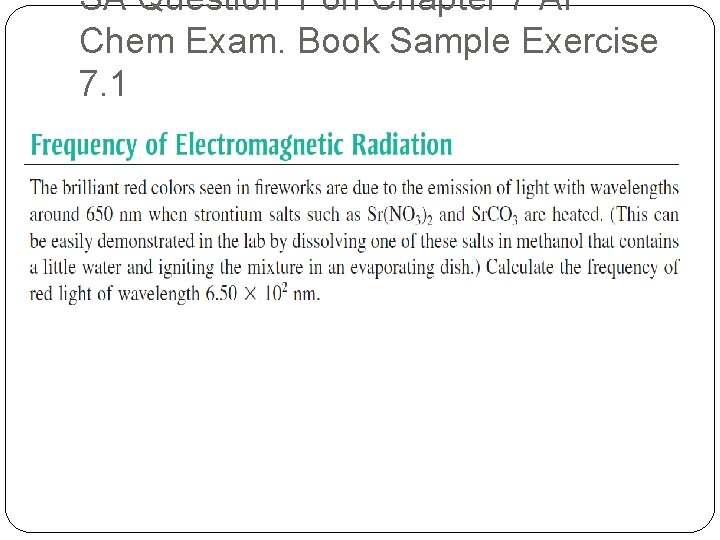SA Question 1 on Chapter 7 AP Chem Exam. Book Sample Exercise 7. 1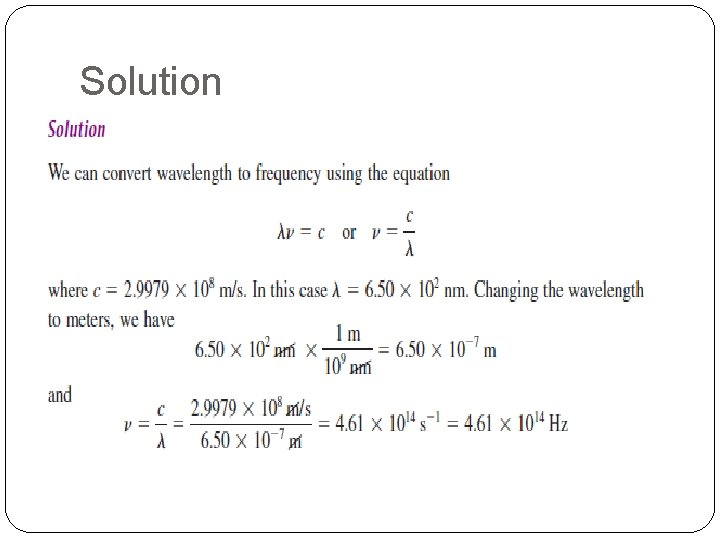Solution7. 2 Nature of Matter �All matter emits an electromagnetic radiation. At first this made scientists think that matter and energy are the same things however in the early 20 th century Max Planck showed again that energy and matter are the same thing �Planck determined that energy can be gained or lost only in whole number multiplies of hv where h is planck’s constant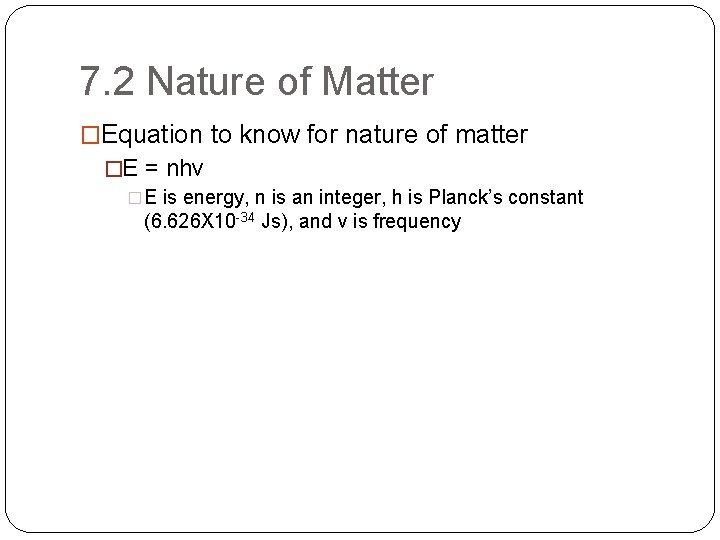7. 2 Nature of Matter �Equation to know for nature of matter �E = nhv �E is energy, n is an integer, h is Planck’s constant (6. 626 X 10 -34 Js), and v is frequency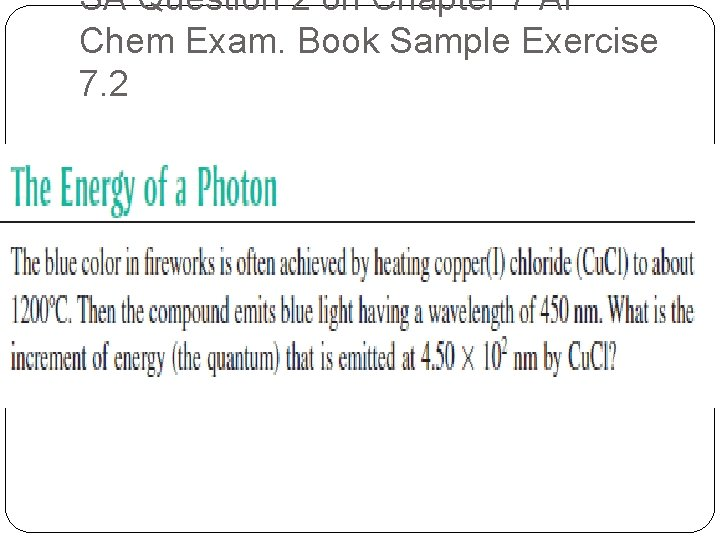SA Question 2 on Chapter 7 AP Chem Exam. Book Sample Exercise 7. 2Solution7. 11 The Aufbau Principal and the Periodic Table �Electron configuration �7. 11 The Aufbau Principal and the Periodic Table �What is the electron configuration of stromium? Draw a picture of how electrons move around the nucleus.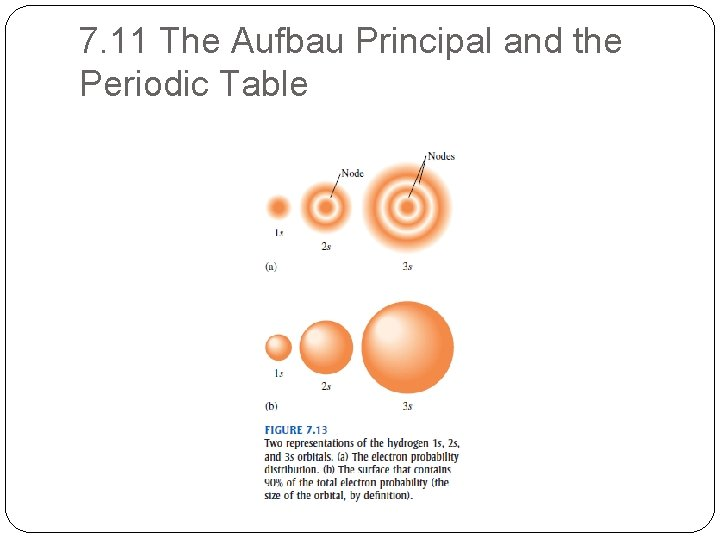7. 11 The Aufbau Principal and the Periodic Table7. 11 The Aufbau Principal and the Periodic Table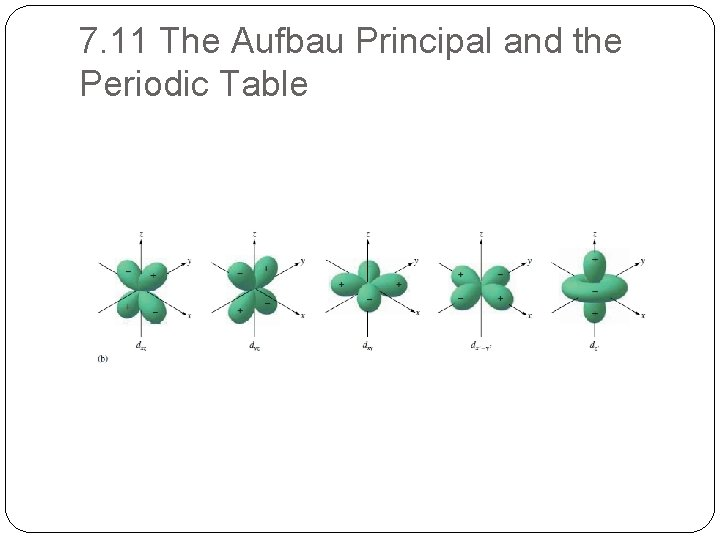7. 11 The Aufbau Principal and the Periodic Table7. 11 The Aufbau Principal and the Periodic Table7. 11 The Aufbau Principal and the Periodic Table �Aufbau Principle: As protons are added one by one to the nucleus to build up the elements, electrons are similar added to these hydrogenlike orbital's. �Hund’s rule: the lowest energy configuration for an atom is the one having the maximum number of unpaired electrons.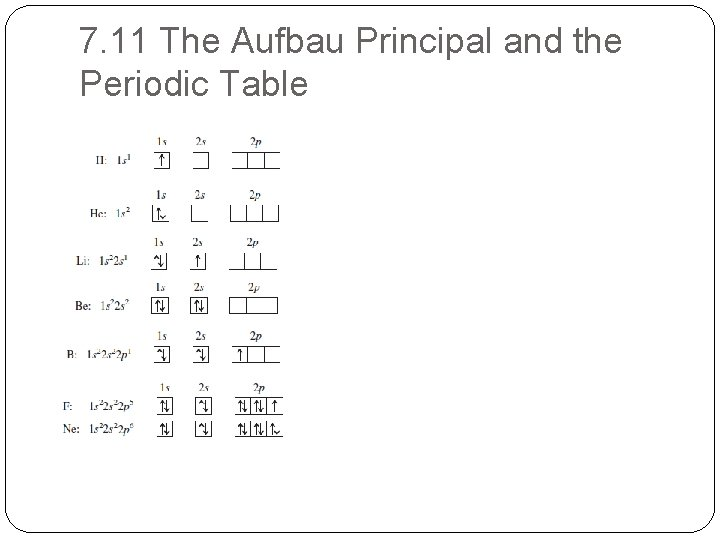7. 11 The Aufbau Principal and the Periodic Table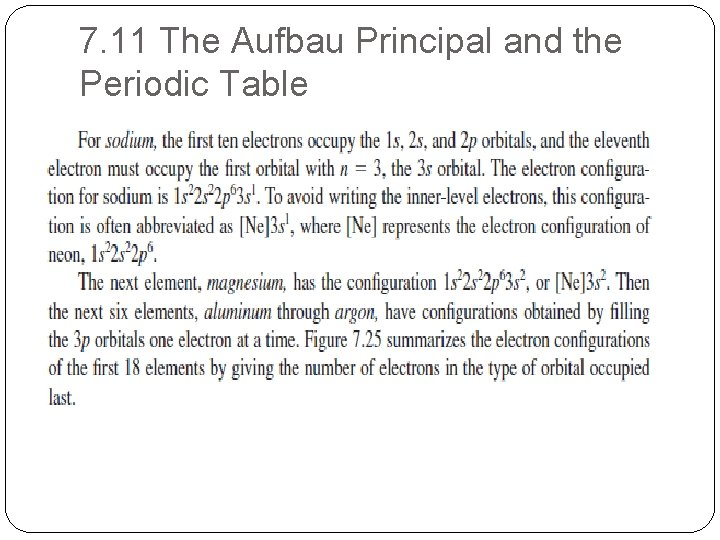7. 11 The Aufbau Principal and the Periodic Table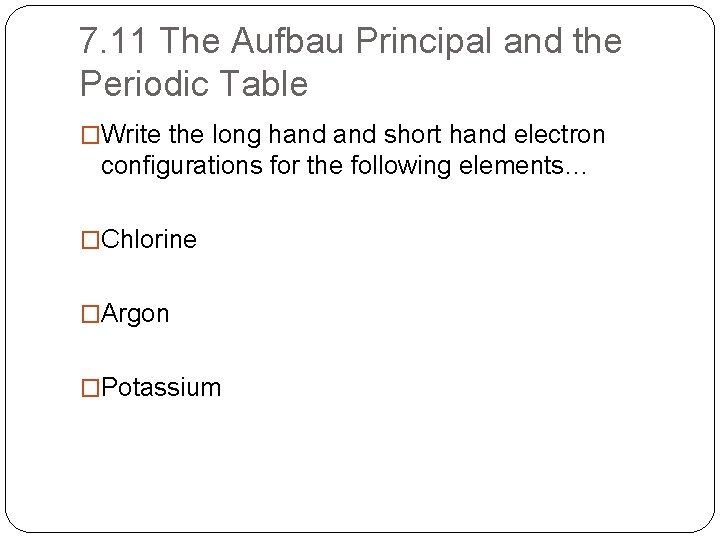7. 11 The Aufbau Principal and the Periodic Table �Write the long hand short hand electron configurations for the following elements… �Chlorine �Argon �Potassium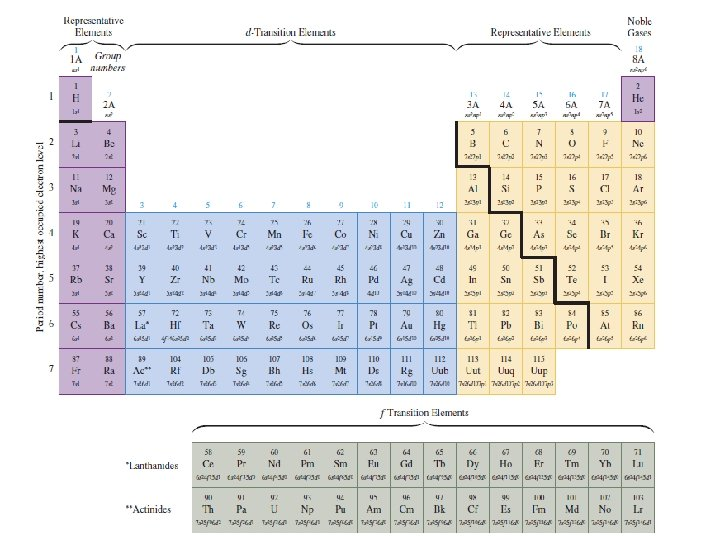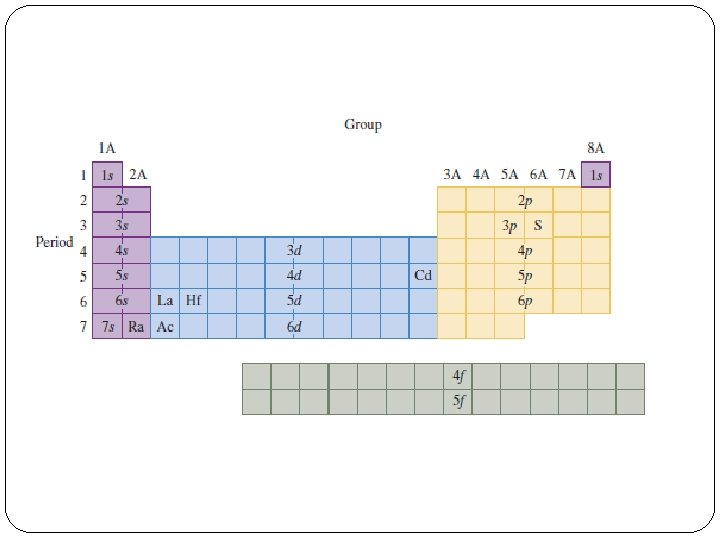SA Question 3 on Chapter 7 Test �Know where the s, d, p, and f block elements are and the numbers associated with them. Hence be able to label the periodic table in last slide. �Be able to identify an element based on the configuration number �i. e. 4 s 2 = Calcium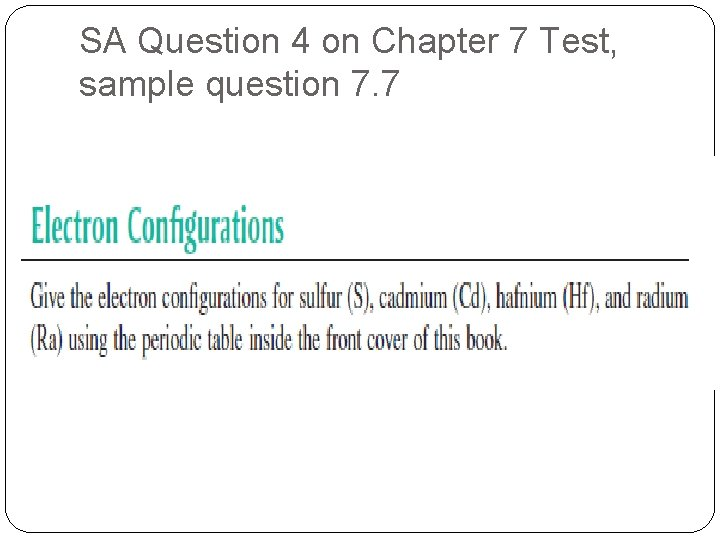SA Question 4 on Chapter 7 Test, sample question 7. 7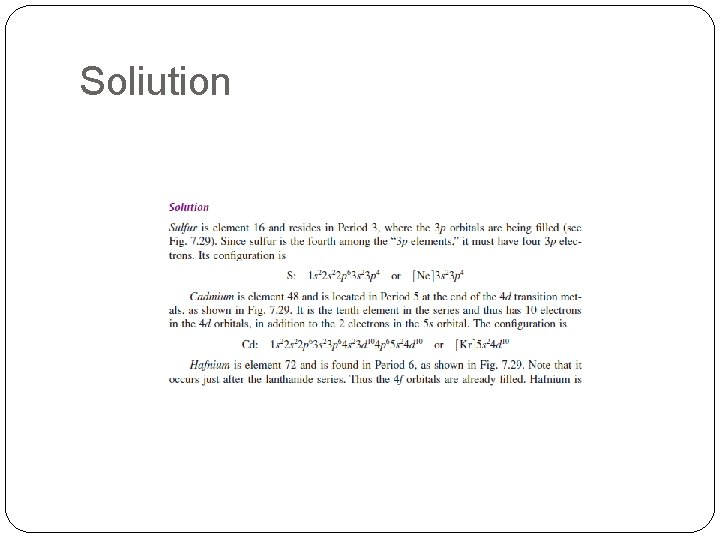SoliutionSolution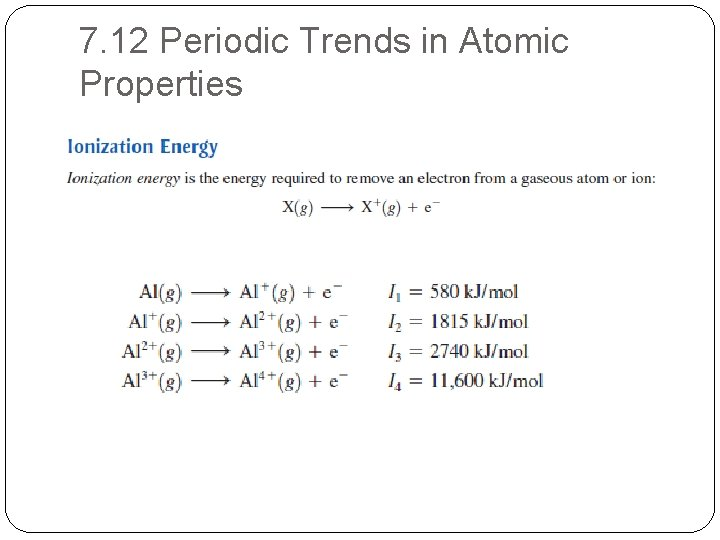7. 12 Periodic Trends in Atomic Properties7. 12 Periodic Trends in Atomic Properties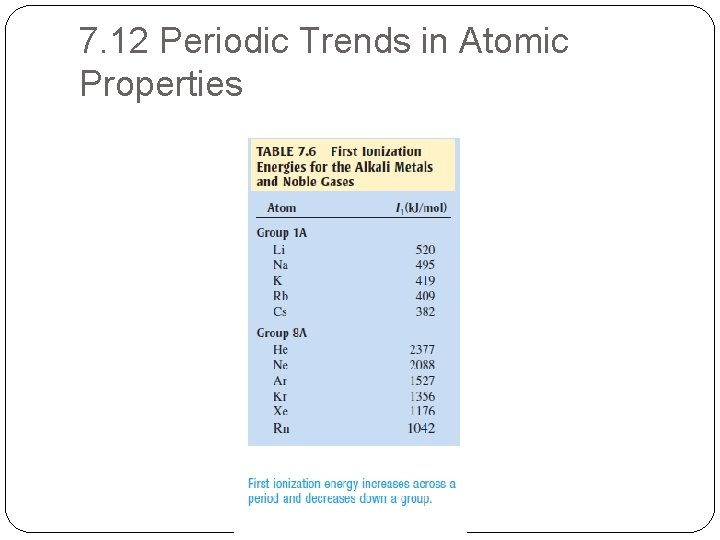7. 12 Periodic Trends in Atomic Properties7. 12 Periodic Trends in Atomic Properties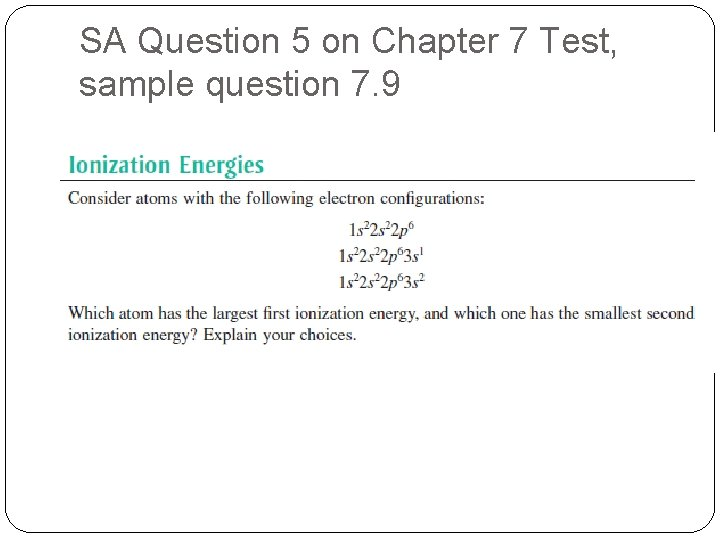SA Question 5 on Chapter 7 Test, sample question 7. 9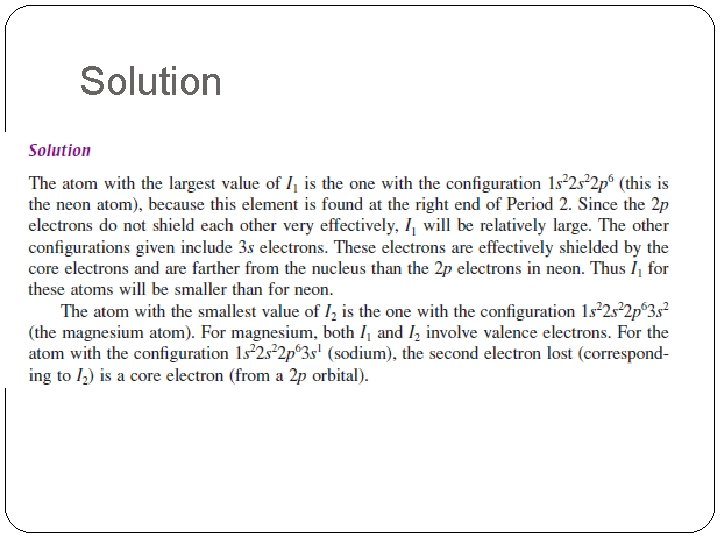Solution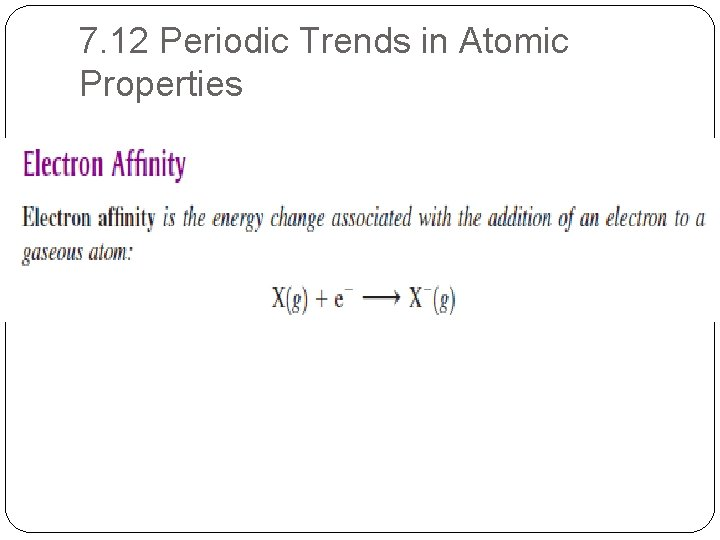7. 12 Periodic Trends in Atomic Properties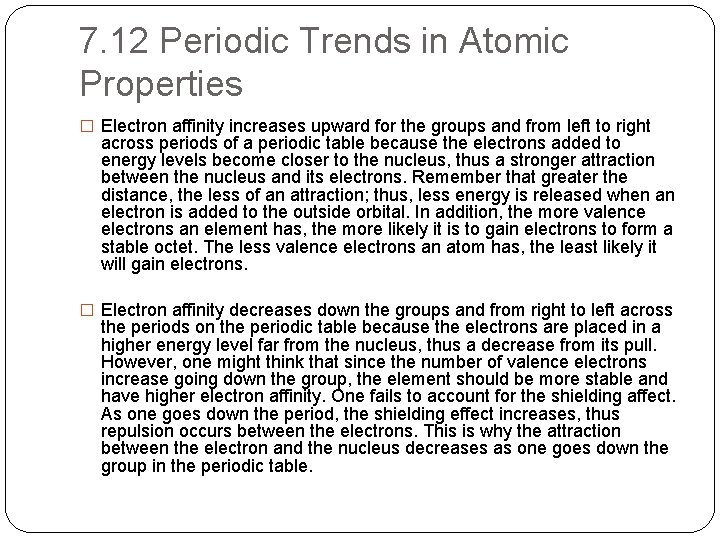7. 12 Periodic Trends in Atomic Properties � Electron affinity increases upward for the groups and from left to right across periods of a periodic table because the electrons added to energy levels become closer to the nucleus, thus a stronger attraction between the nucleus and its electrons. Remember that greater the distance, the less of an attraction; thus, less energy is released when an electron is added to the outside orbital. In addition, the more valence electrons an element has, the more likely it is to gain electrons to form a stable octet. The less valence electrons an atom has, the least likely it will gain electrons. � Electron affinity decreases down the groups and from right to left across the periods on the periodic table because the electrons are placed in a higher energy level far from the nucleus, thus a decrease from its pull. However, one might think that since the number of valence electrons increase going down the group, the element should be more stable and have higher electron affinity. One fails to account for the shielding affect. As one goes down the period, the shielding effect increases, thus repulsion occurs between the electrons. This is why the attraction between the electron and the nucleus decreases as one goes down the group in the periodic table.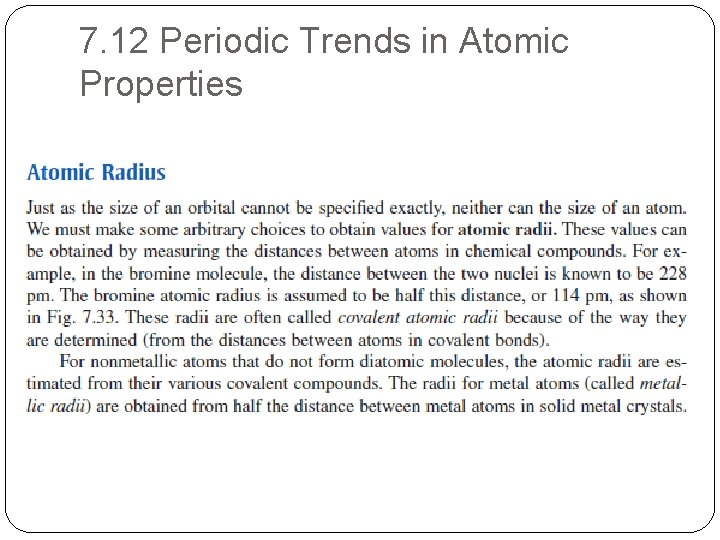7. 12 Periodic Trends in Atomic Properties7. 12 Periodic Trends in Atomic PropertiesSA Question 6 on Chapter 7 Test, sample question 7. 9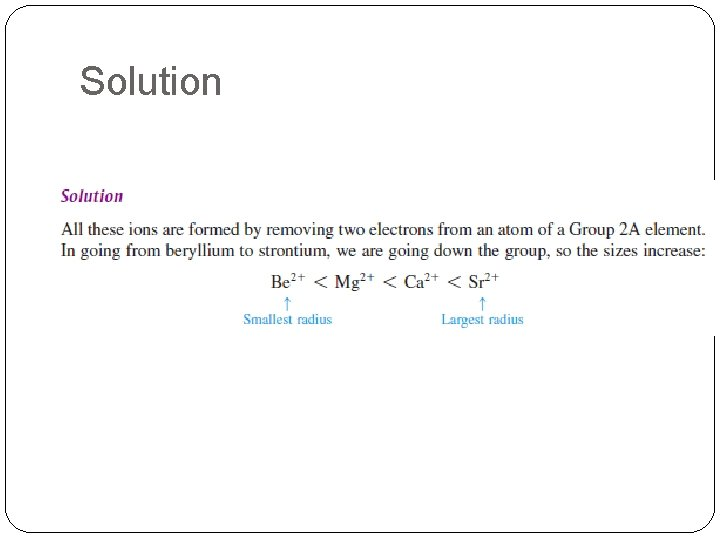Solution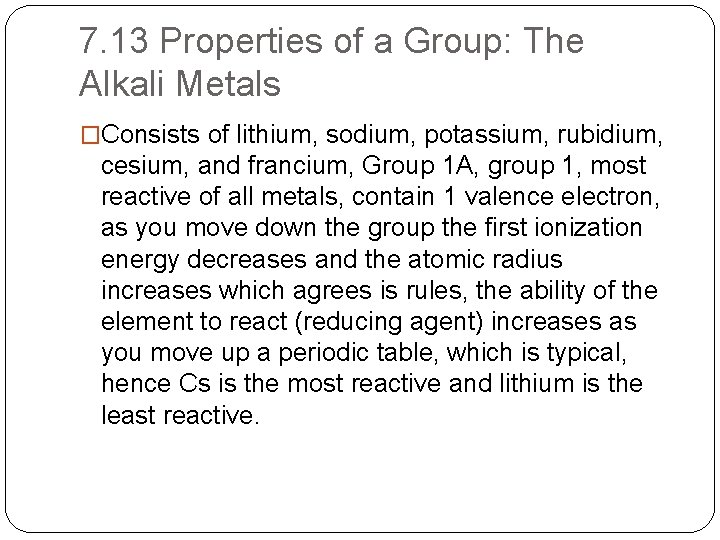7. 13 Properties of a Group: The Alkali Metals �Consists of lithium, sodium, potassium, rubidium, cesium, and francium, Group 1 A, group 1, most reactive of all metals, contain 1 valence electron, as you move down the group the first ionization energy decreases and the atomic radius increases which agrees is rules, the ability of the element to react (reducing agent) increases as you move up a periodic table, which is typical, hence Cs is the most reactive and lithium is the least reactive.